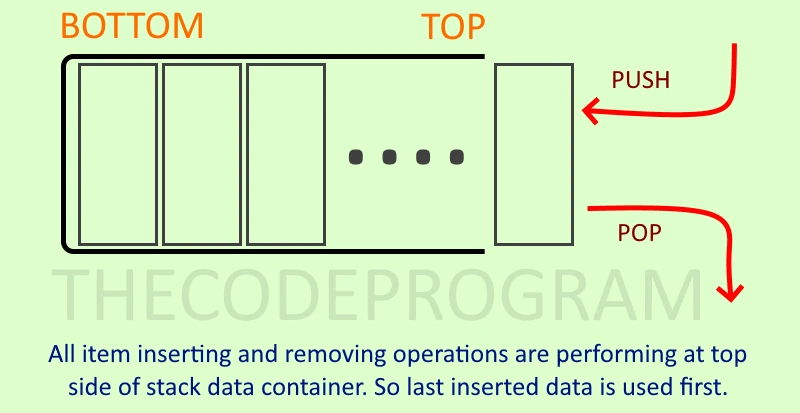## Explanation of QStack and How to use in QT C++

Let's get started.

Firstly, We need to know what Stack Data Structure is
Stack data structure is a linear type data container which follows Last In First Out principle (LIFO). This principle makes the container as Last inserted element will be out first.

Below image you can see the example schematic of Stack Data Structure.Generally, Stack data structure has some basic functions:
• push(...) : push() method insert new item to at the top of stack container.
• pop() : pop() method remove the topmost element of container and return it.
• peek() : It return the topmost element with no removing
• isEmpty() : It controls the stack container is empty
• isFull() : It controls the stack container is full.

Stack container class is a generic class. It implements the Stack data structure to programming language. In Qt framework we see it as QStack class and it inherits QVector class to store datas. QStack class add functionality to manage datas which kept with QVector. So some of functions belong to QVector class directly like isEmpty. We can use all public functions of QVector class according to inheritance.

Now let's make examples about QStack container class in Qt.

Firstly make sure below line is in .pro file:
``````
QT += core
``````
And below library is included by related class which QStack will be used by:
``````
#include <QStack>
``````
Below code block we will see pushing and popping.
``````
QStack<QString> stack;
stack.push("The");
stack.push("Code");
stack.push("Program");
while (!stack.isEmpty())
cout << stack.pop().toLatin1().constData() << endl;
``````
Program output will be
``````
Program
Code
The
``````

As you can see inserting order is reversed. Last inserted element popped out first. It means Last In First Out principle is occured in here.

Now let's take a look at swap function. With swap function we can exchange all elements of two stack containers. Below code block you will see the usage and output.

``````
QStack<int> stack1;
QStack&lt;int&gt; stack2;

stack1.push(1);
stack1.push(2);
stack1.push(3);

stack2.push(4);
stack2.push(5);
stack2.push(6);

stack1.swap(stack2);

cout << "After Stack1" << endl;
while (!stack1.isEmpty())
cout << stack1.pop() << endl;

cout << "After Stack2" << endl;
while (!stack2.isEmpty())
cout << stack2.pop() << endl;
``````
Program output will be below.
``````
After Stack1
6
5
4
After Stack2
3
2
1
``````

As you can see we inserted the 1,2,3 elements into first stack and we swapped. As result we have seen the 4,5,6 inside of it.

QStack container class inherits QVector. So we have some additional methods to get some specifications of our container.
• size : It gets the element number of container.
• isEmpty : It is a general Stack data structure function and it returns is container empty.
• contains : It returns the specified element is exist or not.
• front : It returns the most bottom element.
• clear: It clear the container.
Below code block you will see example of above functions:
``````
QStack<QString> s;
s.push("Burak");
s.push("Hamdi");
s.push("Tufan");

cout << "Number of elements : " << s.size() << endl;
cout << "Is Empty : " << s.isEmpty() << endl;
cout << "Is Stack contains -> Burak : " << s.contains("Burak") << endl;
cout << "Is Stack contains -> The : " << s.contains("The") << endl;
cout << "Front element : " << s.front().toLatin1().constData() << endl;

s.clear();
cout << endl;
cout << "After clean : " << endl;
cout << "Number of elements : " << s.size() << endl;
cout << "Is Empty : " << s.isEmpty() << endl;
``````
Program output
``````
Number of elements : 3
Is Empty : 0
Is Stack contains -> Burak : 1
Is Stack contains -> The : 0
Front element : Burak

After clean :
Number of elements : 0
Is Empty : 1
``````

You can reach the example application source code on Github via : https://github.com/thecodeprogram/TheSingleFiles/blob/master/QtStackExample.cpp

Burak Hamdi TUFAN

29/10/2020

27/02/2021

05/04/2021